# GED Math : Statistics

## Example Questions

### Example Question #81 : Calculations

Evaluate the mean of the following numbers: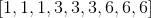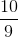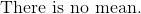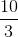Explanation:

The mean is the average of the numbers.  Do not confuse mean with the mode!

Sum all the numbers and divide the total by the amount of numbers in the data set.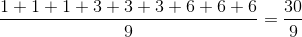Reduce the fraction.

The answer is:### Example Question #26 : Mean

Determine the mean of the numbers: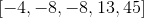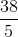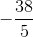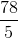Explanation:

The mean is the average of all the numbers in the given data set.

Sum all the numbers and divide the quantity by five.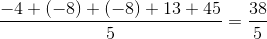The answer is:### Example Question #81 : Statistics

Find the mean of the numbers: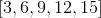Explanation:

The mean is the average of all the numbers given in the data set.

Add all the numbers and divide by five.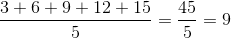The answer is:### Example Question #28 : Mean

Identify the mean: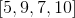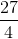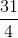Explanation:

The mean is the average of all the numbers given.

Add all the numbers and divide the total by four.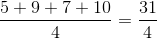The mean is:### Example Question #82 : Calculations

Find the mean of the following data set: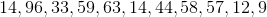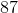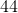Explanation:

Find the mean of the following data set:Now, to find the mean we need to find the average.

Find the average via the following formula: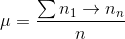What this means is that we will sum all our terms in the numerator, and then divide by the total number of terms (n).

We have 11 terms, so n=11

Let's sum our terms: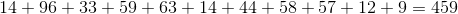Next, divide by 11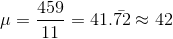So, we can say our answer is 42.

### Example Question #21 : Mean

Find the mean of the following data set: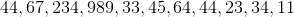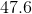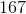Explanation:

Find the mean of the following data set:To find the mean of a series of numbers, simply find the average.

This means that we will sum up our terms, and then divide by the total number of terms in our series.

In this case, we have 11 terms, so we get the following: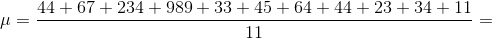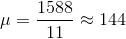So, our mean is 144

### Example Question #31 : Mean

Find the mean of the following data set: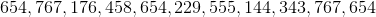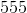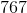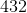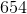Explanation:

Find the mean of the following data set:To find the mean, sum up your terms and divide by the number of terms.

We have 11 terms, so that will be our numerator.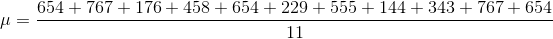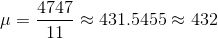### Example Question #31 : Mean

The sales of a construction supply company are shown for the first six months of a year: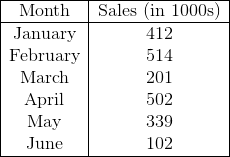In thousands, what is the mean dollar amount of sales for these six months?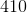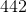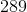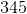Explanation:

Recall how to find the mean: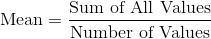Start by finding the sum of all the values given: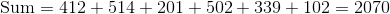Since we have six months, the number of values is.

Now, find the mean: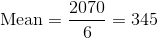### Example Question #33 : Mean

The following chart shows the pounds of strawberries harvested over the span of a week on a farm: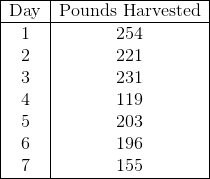What is the difference between the median and the mean of the weights?Explanation:

To find the median, start by placing the values in numerical order: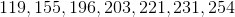The middle term, or the median, of this set is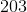.

Next, recall how to find the mean of a set of values: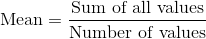Thus, the mean for this set of values is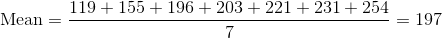The difference between the median and the mean is then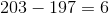### Example Question #34 : Mean

The following chart shows the pounds of strawberries harvested over the span of a week on a farm:How many pounds of strawberries must be harvested on the eighth day for the average pounds of strawberries harvested each day to increase topounds?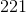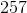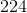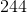Explanation:

Recall how to find the mean:The question gives us the mean and the number of values. Letbe the number of pounds that must be harvested on the eighth day, and then we can write the following equation and solve: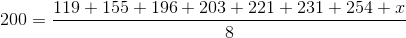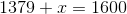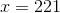pounds must be harvested on the eighth day in order for the average pounds of strawberries harvested to increase the desired amount.

### All GED Math Resources